Geometric Mean - Measures of Central Tendency, Business Mathematics & Statistics

# Geometric Mean - Measures of Central Tendency, Business Mathematics & Statistics - Business Mathematics and Statistics - B Com

GEOMETRIC MEAN (G. M.) Definition. :
The geometric mean (G) of the n positive values x1 , x2 , x3 ………….xn is the nth root of the product of the values i.e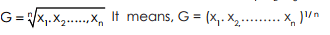Now taking logarithms on both sides, we find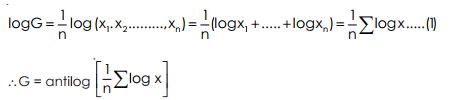Thus, from formula (1) we find that the logarithm of the G. M. of x1 , x2 ….., xn = A.M. of logarithms of x1 , x2 , …..., xn .

Properties :
1. The product of n values of a variate is equal to the nth power of their G. M. i.e., x1 , x2 , ……, xn = Gn (it is clear from the definition)]
2. The logarithm of G. M. of n observations is equal to the A.M. of logarithms of n observations. [Formula (1) states it]

3. The product of the ratios of each of the n observations to G. M. is always unity. Taking G as geometric mean of n observations x1 , x2 , ……., xn the ratios of each observation to the geometric mean are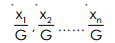By definition,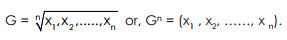Now the product of the ratios.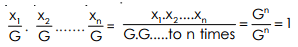4. If G1 , G2……, are the geometric means of different groups having observations n1 , n2………respectively, then the G. M. (G) of composite group is given by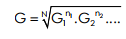where N = n1 + n2 + …..i.e., log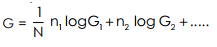Example 15 : Find the G. M. of the number 4, 12, 18, 26.

Solution :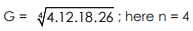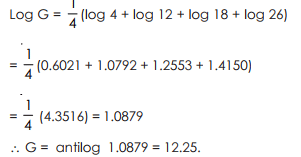Weighted Geometric Mean : If f1 , f2 , f3……f n are the respective frequencies of n variates x1 , x2 , x3 ,…….xn , then the weighted G. M. will be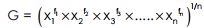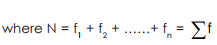Now taking logarithm.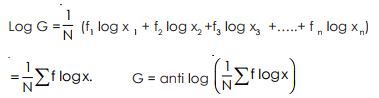Steps to calculate G. M.
1. Take logarithm of all the values of variate x.
2. Multiply the values obtained by corresponding frequency
3. Find f log x and divide it by f , i.e., calculate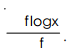4. Now antilog of the quotient thus obtained is the required G. M. The idea given above will be clear from the following example.

Example 16 : Find (weighted) G. M. of the table given below : ––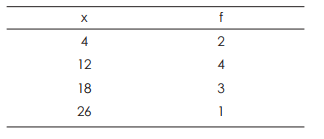Solution :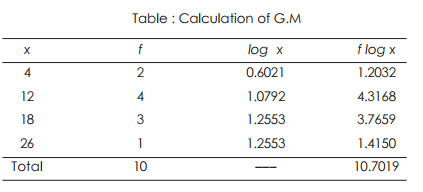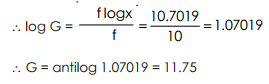(i) It is not influenced by the extreme items to the same extent as mean.
(ii) It is rigidly defined and its value is a precise figure.
(iii) It is based on all observations and capable of further algebraic treatment.
(iv) It is useful in calculating index numbers.

(i) It is neither easy to calculate nor it is simple to understand.
(ii) If any value of a set of observations is zero, the geometric mean would be zero, and it cannot be determined.
(iii) If any value is negative, G. M. becomes imaginary. [Use. It is used to find average of rates of changes.]

The document Geometric Mean - Measures of Central Tendency, Business Mathematics & Statistics | Business Mathematics and Statistics - B Com is a part of the B Com Course Business Mathematics and Statistics.
All you need of B Com at this link: B Com

115 videos|142 docs

## FAQs on Geometric Mean - Measures of Central Tendency, Business Mathematics & Statistics - Business Mathematics and Statistics - B Com

 1. What is the formula for calculating the geometric mean?Ans. The formula for calculating the geometric mean is to multiply all the numbers together and then take the nth root of the product, where n is the total number of values. Mathematically, it can be represented as: Geometric Mean = (x1 * x2 * x3 * ... * xn)^(1/n).
 2. How is the geometric mean useful in business mathematics and statistics?Ans. The geometric mean is useful in business mathematics and statistics because it provides a measure of central tendency that is appropriate for data sets with skewed distributions or involving ratios and rates. It is commonly used to calculate average growth rates, investment returns, or to compare performance across different time periods or entities.
 3. Can the geometric mean be used with negative numbers?Ans. No, the geometric mean cannot be used with negative numbers as it involves taking the nth root of the product of values. Since negative numbers do not have real roots for even values of n, the geometric mean is only applicable to data sets with positive values.
 4. How does the geometric mean differ from other measures of central tendency, such as the mean or median?Ans. The geometric mean differs from other measures of central tendency in that it is specifically designed to handle data sets with skewed distributions or involving ratios and rates. Unlike the arithmetic mean, it does not give equal weight to each value, but instead emphasizes the contribution of smaller values. Additionally, it is not affected by extreme values like the median.
 5. Can the geometric mean be used to compare data sets with different units of measurement?Ans. Yes, the geometric mean can be used to compare data sets with different units of measurement. Since it involves taking the nth root of the product of values, the resulting value is a ratio or rate that is independent of the original units. This makes it a useful tool for comparing growth rates, investment returns, or other performance indicators across different entities or time periods.

115 videos|142 docsExplore Courses for B Com examSignup to see your scores go up within 7 days! Learn & Practice with 1000+ FREE Notes, Videos & Tests.
10M+ students study on EduRev
Track your progress, build streaks, highlight & save important lessons and more!
Related Searches

,

,

,

,

,

,

,

,

,

,

,

,

,

,

,

,

,

,

,

,

,

,

,

,

;